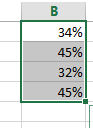# How to Add Percentage Symbol without Multiplying 100 in Excel

This post will guide you how to add a percentage sign without multiplying by 100 in cells in Excel. How do I add percentage symbol only in Excel without multiplying 100. How to show % sign without multiplying with number 100 for multiple cells in Excel.

## Add Percentage Symbol without Multiplying 100

Generally, when you add a percentage sign to a number in Excel, it will multiply the number by 100 firstly, and then insert the percentage symbol behind the number. So if you only want to add a percentage single behind the number, you can do the following steps:

Assuming that you have a list of data in range B1:B4 which contain numbers, you want to add percentage sign to those numbers.

#1 select one blank cell, such as: C1

#2 type the following formula in cell C1 and press Enter key in your keyboard, and then press drag the AutoFill Handle over to cell C4 to apply this formula.

=B1/100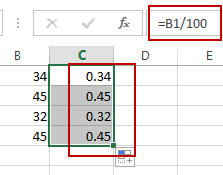#3 select range C1:C4, and go to HOME tab, click percentage button under Number group.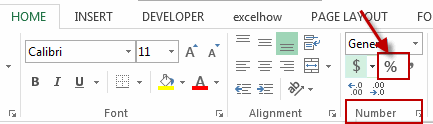#4 all numbers in range C1:C4 will be added a percentage sign without multiplying 100.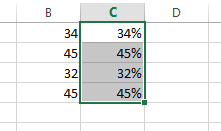Or you can do the following steps to achieve the same result of add percentage symbol without multiplying 100 for numbers in range B1:B4.

#1 select those numbers in new column, and then right click on it, and select Format Cells from the popup menu list. And the Format Cells dialog will open.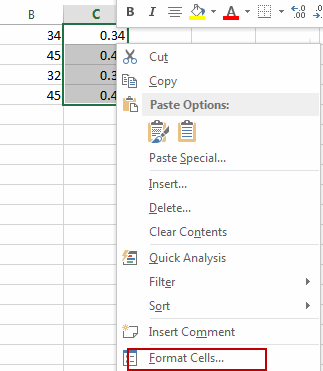#2 click Custom under Category list box, and type in “0\%” in Type text box, and then click OK button.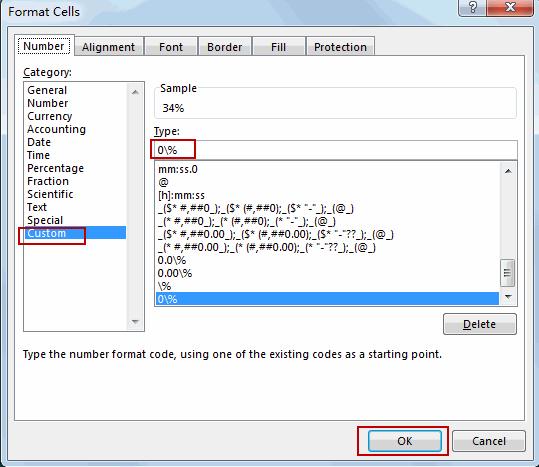#3 only percentage symbol without multiplying 100 will be added in those numbers.